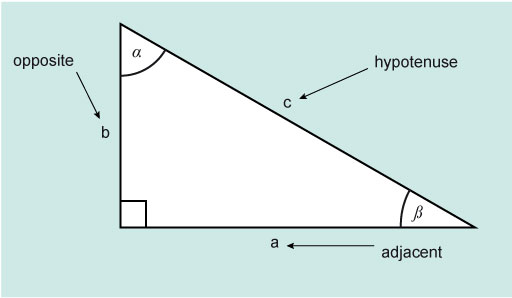Science, Maths & Technology

### Become an OU studentMathematics for science and technology

Start this free course now. Just create an account and sign in. Enrol and complete the course for a free statement of participation or digital badge if available.

# 2 Sine, cosine and tangent

Sin, cos and tan are the commonly used abbreviations for each function. So:

• Sin = sine
• Cos = cosine
• Tan = tangent

Sine is pronounced as ‘sign’ and cosine as ‘co-sign’.

Based on the triangle in Figure 2 these functions are defined as follows:Figure 2 A right angle triangle

There is an easy way to remember this: SOHCAHTOA (pronounced sock -ah- toe-a).

Using these functions, and Pythagoras theorem, if you know two sides or a side and an angle for a right-angled triangle, then you can find the other side, sides or angle as required.

## Activity 2 Sine and cosine values between 0° and 90°

Making sure that your calculator is still set to degrees, work out these values for sine and cosine.

 1 Sin 0° 2 Cos 0° 3 Sin 10° 4 Cos 10° 5 Sin 25° 6 Cos 25° 7 Sin 45° 8 Cos 45° 9 Sin 70° 10 Cos 70° 11 Sin 90° 12 Cos 90°

Angle/degreesSineCosine
001
100.17360.9848
250.42260.9063
450.70710.7071
700.93970.3420
9010

Perhaps you noticed that all the values for sine and cosine seem to lie between 0 and 1 for angles between 0° and 90°.

You may have also observed that as the values for sine increase, the values for cosine decrease.

In fact, for any angle between 0° and 90° the values for sine and cosine lie between 0 and 1.

These two graphs (Figures 3 and 4) show the shape of the sine and cosine functions between 0 and 360°. From this you can see that both functions stay within –1 and +1.Figure 3 The sine functionFigure 4 The cosine function

As shown in Figure 5 the tangent function is not similar in the way that cosine and sine are but forms its own repeating pattern.Figure 5 The tangent function

Now it is time to see how you can use these functions with right-angled triangles.